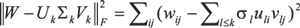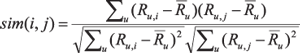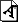# Who Rated What? A Recommender System Benchmark Winner Report

The KDD (Knowledge Discovery in Databases) Cup is the world's most prestigious data-mining competition, and is held each year in conjunction with the conference of the ACM Special Interest Group for Knowledge Discovery in Databases (ACM SIGKDD). Last year's competition saw participants from leading international institutions such as IBM research, Neo Metrics and Inductis.

The competition was organized over a unique data set of great interest to the research community. In October 2006 Netflix provided over 100 million ratings from over 480 000 randomly chosen anonymous customers on nearly 18 000 movie titles. KDD Cup 2007 focused on predicting aspects of movie-rating behaviour. In Task 1 'Who Rated What in 2006?', the goal was to predict which users rated which movies in 2006; for Task 2 'How Many Ratings in 2006?', participants had to predict the number of additional ratings of movies.Figure 1: Sample time series for the number of ratings of a movie with related events

In our winning prediction method for Task 1, we used a combination of the following major methods from three different areas of applied mathematics, listed in ascending/descending? order of accuracy:

• linear algebra: a low-rank approximation of the known user/movie pair matrix
• statistics: movie-movie similarity values corrected by confidence interval
• data-mining algorithms: association rules obtained by frequent sequence mining of user ratings considered as ordered item sets.

By combining the predictions by linear regression, we reached first place with a prediction with root mean squared error 0.256, which can be further improved to 0.245 by extended experimentation with association rules. The runner-up result from Neo Metrics was 0.263. Compare this with a pure all-zeroes prediction giving 0.279, and the difficulty of the task is evident.

In our first method based on linear algebra, we used the full 01 matrix of all known ratings for training, and the rank k approximation of this matrix yielded our prediction. A rank k approximation can be considered as a prediction based on k independent factors. While in practice the explanation of the factors is never obvious, we may think of family movies, thrillers, comedies etc as factors, and model users as a mixture of such interests. The Singular Value Decomposition (SVD) of a rank r matrix W is given by W = UTΣV with U an m × r, Σ a r × r and V an n × r matrix such that U and V are unitary. By a classical result of linear algebra, the Eckart-Young theorem, the best rank k approximation of W with respect to the Frobenius norm is given by:Here Uk is an m × k and Vk is an n × k matrix containing the first k columns of U and V and the diagonal Σk containing first k entries of Σ where uli and vlj denotes the strength of user i and movie j in factor l, respectively, while σl is the factor importance weight. Since we observed overfitting for larger number of dimensions, we used the 10-dimensional approximation. In our implementation we used the Lánczos code of the publicly available solver svdpack in which we abstracted data access within the implementation to computing the product of a vector with either the input matrix or its transpose in order to handle the matrix with 100 million non-zeroes.

Our item-item similarity-based recommender computes the adjusted cosine similarity based not just on the existence of the ratings wij, but also their values Rij in the range of one to five stars according to:Here is the average of the ratings of user u and summations are for all users u who rated both j and j0. Since the number of such users can sometimes be very small, we replaced the above value by its lower 95% confidence interval of the adjusted cosine similarity obtained by Fisher's r-to-z transform using 1/(njj0 - 3) for the squared standard deviation:

z = (ln(1 + r) - ln(1 - r))/2.

In our third method, we used association rules for movies mi of form m1, , ms --> m where the number of users who rated {m1, ... , ms, m} are above the prescribed minimum support. The confidence of this rule is the ratio of users who rated {m1, ... , ms, m} versus just {m1, ... , ms}.

In combination with linear regression, low-rank approximation performed best, a phenomenon considered to be more or less 'fact' by the movie ratings recommender community. Association rules performed very close to a relatively carefully tuned item-item recommender. We stress here that tuning predictions based on association rules is a very time-consuming and resource-heavy task and our method is far from being the result of exhaustive experimentation. The main lesson learned is probably the fact that very different data-mining techniques catch very similar patterns in the data, making it increasingly difficult to improve prediction quality beyond a certain point.

Our results can be applied in an online or mobile service for recommending books, news articles, RSS feed items or arbitrary products given rating or text description. In the current phase we are building a framework that is capable of integrating our existing algorithms with a flexible framework to serve a wide range of recommender applications.

Data Mining and Web Search Group: http://datamining.sztaki.hu
The KDD Cup 2007: http://www.cs.uic.edu/Netflix-KDD-Cup-2007/
The full research report:
E-mail: benczursztaki.hu